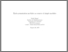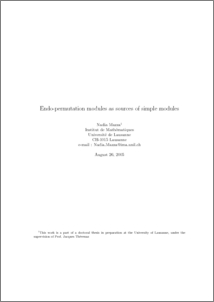# Endo-permutation modules as sources of simple modules.

Mazza, Nadia (2003) Endo-permutation modules as sources of simple modules. Journal of Group Theory, 6 (4). pp. 477-497. ISSN 1435-4446Preview
PDF (endo-permutation-modules-as-sources-of-simple-modules.pdf)
endo-permutation-modules-as-sources-of-simple-modules.pdf

The source of a simple $kG$-module, for a finite $p$-solvable group $G$ and an algebraically closed field $k$ of prime characteristic $p$, is an endo-permutation module (see~\cite{Pu1} or~\cite{Th}). L. Puig has proved, more precisely, that this source must be isomorphic to the cap of an endo-permutation module of the form $\bigotimes_{Q/R\in\cal S}\Ten^P_Q\Inf^Q_{Q/R}(M_{Q/R})$, where $M_{Q/R}$ is an indecomposable torsion endo-trivial module with vertex $Q/R$, and $\cal S$ is a set of cyclic, quaternion and semi-dihedral sections of the vertex of the simple $kG$-module. At present, it is conjectured that, if the source of a simple module is an endo-permutation module, then it should have this shape. In this paper, we are going to give a method that allow us to realize explicitly the cap of any such indecomposable module as the source of a simple module for a finite $p$-nilpotent group.View Item